# Class 11 Maths NCERT Solutions for Chapter 10 Straight Lines Miscellaneous Exercise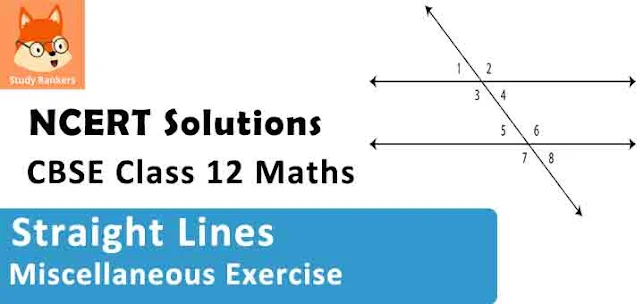### Straight Lines Miscellaneous Exercise Solutions

1. Find the values of k for which the line (k–3) x – (4 – k2) y + k2 –7k + 6 = 0 is

(a) Parallel to the x-axis,
(b) Parallel to the y-axis,
(c) Passing through the origin.

Solution

The given equation of line is
(k – 3)x – (4 – k2)y + k2 – 7k + 6 = 0 …(1)

(a) If the given line is parallel to the x-axis, then
Slope of the given line = Slope of the x-axis
The given line can be written as
(4 – k2) y = (k – 3) x + k2 – 7k + 6 = 0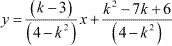, which is of the form y = mx + c.
∴ Slope of the given line = (k - 3)/(4 - k2
Slope of the x - axis = 0
∴ (k -3)/(4 - k2) = 0
⇒ k - 3 = 0
⇒ k = 3
Thus, if the given line is parallel to the x-axis, then the value of k is 3.

(b) If the given line is parallel to the y-axis, it is vertical. Hence, its slope will be undefined.
The slope of the given line is (k -3)/(4 - k2
Now, (k -3)/(4 - k2) is undefined at k2 = 4
k2 = 4
⇒ k = ± 2
Thus, if the given line is parallel to the y-axis, then the value of k is ±2.

(c) If the given line is passing through the origin, then point (0, 0) satisfies the given equation of  line.
(k - 3)(0) - (4 - k2) (0) + k2 - 7k + 6 = 0
⇒ k2 - 7k + 6 = 0
⇒ k2 - 6k - k + 6 = 0
⇒ (k - 6)(k -1) = 0
⇒ k = 1 or 6
Thus, if the given line is passing through the origin, then the value of k is either 1 or 6.

2. Find the values of q and p, if the equation x cos q + y sin q = p is the normal form of the line √3x + y + 2 = 0.

Solution

The equation of the given line is √3x + y + 2 = 0.
This equation can be reduced as
√3x + y + 2 = 0
⇒ -√3x - y = 2
On dividing both sides by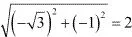we obtain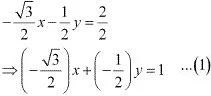On comparing equation (1) to x cos θ + y sin θ = P, we obtain
cos θ = -√3/2 , sin θ = -1/2 , and p = 1
Since the value of sin θ and cos θ are negative, θ = π + π/6 = 7π/6
Thus, the respective values of θ and p are 7π/6 and 1

3. Find the equations of the lines, which cut-off intercepts on the axes whose sum and product are 1 and –6, respectively.

Solution

Let the intercepts cut by the given lines on the axes be a and b.
It is given that
a + b = 1 …(1)
ab = –6 …(2)
On solving equations (1) and (2), we obtain
a = 3 and b = –2 or a = –2 and b = 3
It is known that the equation of the line whose intercepts on the axes are a and b is
x/a + y/b = 1 or bx + ay - ab = 0
Case I: a = 3 and b = -2
In this case, the equation of the line is  -2x + 3y + 6 = 0, i.e., 2x - 3y = 6
Case II: a = -2 and b = 3
In this case, the equation of the line is  3x - 2y + 6 = 0, i.e., -3x + 2y = 6.
Thus, the required equation of the lines are 2x - 3y = 6 and -3x + 2y = 6.

4. What are the points on the y-axis whose distance from the line x/3 + y/4 = 1 is 4 units

Solution

Let (0, b) be the point on the y - axis whose distance from line x/3 + y/4 = 1 is 4 units.
The given line can be written as  4x + 3y - 12 = 0  ...(1)
On comparing equation (1) to the general equation of line Ax + By + C = 0, we obtain A = 4, B = 3 and C = -12.
It is known that the perpendicular distance (d) of a line Ax + By + C = 0 from a point (x1y1) is given by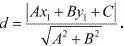Therefore, if (0, b) is the point on the y- axis whose distance from line x/3 + y/4 = 1 is 4 units, then :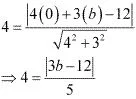⇒ 20 = |3b - 12|
⇒ 20 = ± (3b - 12)
⇒ 20 = (3b - 12) or 20 = -(3b - 12)
⇒ 3b = 20 + 12 or 3b = -20 + 12
⇒ b = 32/3 or b = -8/3
Thus, the required points are (0, 32/3) and (0, -8/3).

5. Find perpendicular distance from the origin to the line joining the points (cosθ, sin θ) and (cosΦ, sin Φ).

Solution

The equation of the line joining the points (cosθ, sinθ) and (cos Φ, sinΦ) is given by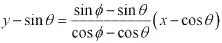y(cosΦ - cos θ) - sin θ(cosΦ - cos θ) = x(sinΦ - sin θ) - cos θ(sinΦ - sin θ)
x(sinθ - sinΦ) + y(cos Φ - cos θ) + cos θ sin Φ - cos θ sinθ - sin θ cosΦ + sinθcosθ = 0
x(sinθ - sinΦ) + y(cos Φ - cos θ) + sin (Φ - θ) =
Ax + By + C = 0, where A = sin θ - sin Φ, B = cos Φ - cos θ, and C = sin(Φ - θ)
It is known that the perpendicular distance (d) of a line Ax + By + C = 0 from a point (x1y1) is given byTherefore, the perpendicular distance (d) of the given line from point  (x1y1) = (0, 0) is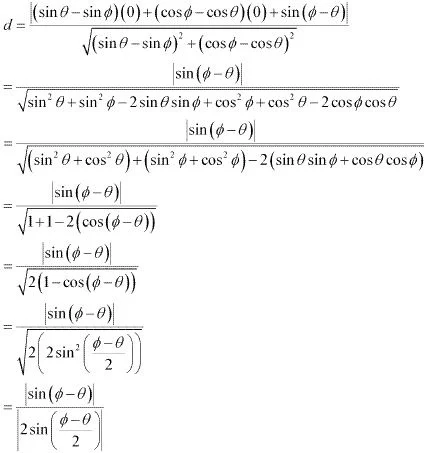6 Find the equation of the line parallel to y-axis and drawn through the point of intersection of the lines x– 7y + 5 = 0 and 3x + y = 0.

Solution

The equation of any line parallel to the y-axis is of the form
x = a …(1)
The two given lines are
x – 7y + 5 = 0 ...(2)
3x + y = 0 …(3)
On solving equations (2) and (3), we obtain x = -(5/22) and y = 15/22
Therefore, (-5/22, 15/22) is the point of intersection of lines (2) and (3).
Since line x = a passes through point (-5/22, 15/22), a = -5/22.
Thus, the required equation of the line is x = -5/22.

7. Find the equation of a line drawn perpendicular to the line  x/4 + y/6 = 1 through the point, where it meets the y - axis.

Solution

The equation of the given line is x/4 + y/6 = 1.
This equation can also be written as 3x + 2y - 12 = 0
y = -(3/2)x + 6, which is of the form y = mx + c
∴ Slope of the given line = -3/2
∴ Slope of the line perpendicular to the given line  = -1/(-3/2) = 2/3
Let the given line intersect the y - axis at (0, y).
On substituting x with 0 in the equation of the given line, we obtain y/6 = 1⇒ y = 6
∴The given line intersects the y-axis at (0, 6).
The equation of the line that has a slope of 2/3 and passes through point (0, 6) is
(y - 6) = (2/3) (x - 0)
⇒ 3y - 18 = 2x
⇒ 2x - 3y + 18 = 0
Thus, he required equation of the line is  2x - 3y + 18 = 0.

8. Find the area of the triangle formed by the lines y – x = 0, x + y = 0 and x – k = 0.

Solution

The equations of the given lines are
y – x = 0 …(1)
x + y = 0 …(2)
x – k = 0 …(3)
The point of intersection of lines (1) and (2) is given by
x = 0 and y = 0
The point of intersection of lines (2) and (3) is given by
x = k and y = –k
The point of intersection of lines (3) and (1) is given by
x = k and y = k
Thus, the vertices of the triangle formed by the three given lines are (0, 0), (k, –k), and (k, k).
We know that the area of a triangle whose vertices are (x1, y1), (x2, y2), and (x3, y3) is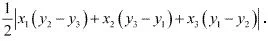Therefore, area of the triangle formed by the three given lines9. Find the value of p so that the three lines 3x + y – 2 = 0, px + 2y – 3 = 0 and 2x – y – 3 = 0 may intersect at one point.

Solution

The equations of the given lines are
3x + y – 2 = 0 …(1)
px + 2y – 3 = 0 …(2)
2x – y – 3 = 0 …(3)
On solving equations (1) and (3), we obtain
x = 1 and y = –1
Since these three lines may intersect at one point, the point of intersection of lines (1) and (3) will also satisfy line (2).
p (1) + 2 (–1) – 3 = 0
⇒ p – 2 – 3 = 0
⇒ p = 5
Thus, the required value of p is 5.

10. If three lines whose equations are y = m1x + c1, y = m2x + c2 and y = m3x + c3 are concurrent, then show that m1(c2 – c3) + m2 (c3 – c1) + m3 (c1 – c2) = 0.

Solution

The equations of the given lines are
y = m1x + c1 …(1)
y = m2x + c2 …(2)
y = m3x + c3 …(3)
On subtracting equation (1) from (2), we obtain
0 = (m2 - m1)x + (c2 - c1
⇒ (m1 - m2)x = c2 - c1
⇒ x = (c2 - c1)/(m1 - m2)
On substituting this value of x in (1), we obtain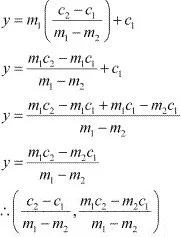is the point of intersection of lines (1) and (2).
It is given that lines (1), (2) and (3) are concurrent. Hence, the point of intersection of lines (1) and (2) will also satisfy equation (3).11. Find the equation of the lines through the point (3, 2) which make an angle of 45° with the line x –2y = 3.

Solution

Let the slope of the required line be m1.
The given line can be written as , which is of the form y = mx + c
∴ Slope of the given line =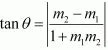It is given that the angle between the required line and line x – 2y = 3 is 45.
We know that if θisthe acute angle between lines l1 and l2 with slopes m1 and mrespectively, then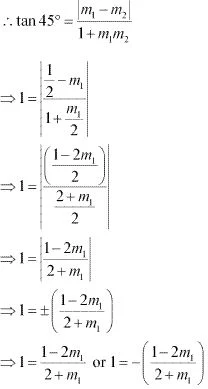⇒ 2 + m1 = 1 - 2m1 or 2 + m1 = -1 + 2m1
⇒ m1 = -1/3 or m1 = 3
Case I: m1 = 3
The equation of the line passing through (3, 2) and having a slope of 3 is:
y – 2 = 3 (x – 3)
⇒ y – 2 = 3x – 9
⇒ 3x – y = 7
Case II: m1 =  -1/3
The equation of the line passing through (3, 2) and having a slope of  -1/3 is :
(y -2) = (-1/3)(x - 3)
⇒ 3y - 6 = -x + 3
⇒ x + 3y = 9
Thus, the equations of the lines are 3x - y = 7 and x + 3y = 9.

12. Find the equation of the line passing through the point of intersection of the lines 4x + 7y – 3 = 0 and 2x– 3y + 1 = 0 that has equal intercepts on the axes.

Solution

Let the equation of the line having equal intercepts on the axes be
x/a + y/a = 1
Or x + y = a ...(1)
On solving equations 4x + 7y - 3 = 0 and 2x - 3y + 1 = 0, we obtain x = 1/13 and y = 5/13 .
∴ (1/13, 5/13) is the point of intersection of the two given lines.
Since equation (1) passes through point (1/13, 5/13),
1/13 + 5/13 = a
⇒ a = 6/13
∴ Equation (1) becomes x + y = 6/13, i.e., 13x + 13y = 6
Thus, the required equation of the line is 13x + 13y = 6.

13. Show that the equation of the line passing through the origin and making an angle θ with the line y = mx + c is y/c = (m ± tan θ)/(1 ± m tan θ)

Solution

Let the equation of the line passing through the origin be y = m1x.
If this line makes an angle of θ with line y = mx + c, then angle θ is given by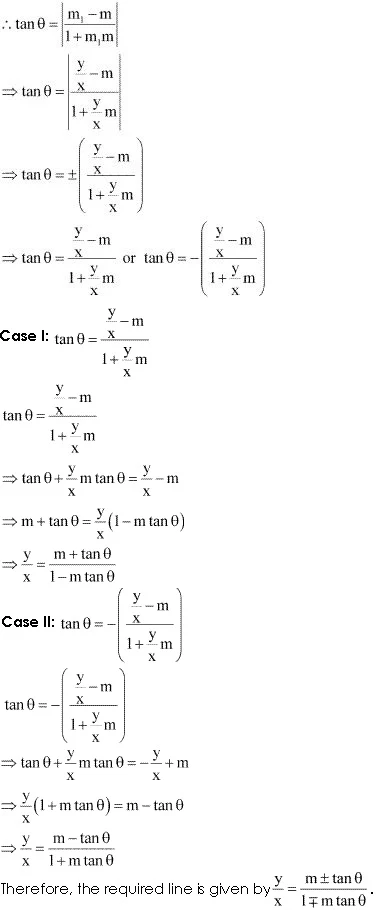14. In what ratio, the line joining (–1, 1) and (5, 7) is divided by the line x + y = 4?

Solution

The equation of the line joining the points (-1, 1) and (5, 7) is given by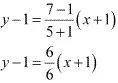x - y + 2 = 0 ...(1)
The equation of the given line is
x + y – 4 = 0 …(2)
The point of intersection of lines (1) and (2) is given by
x = 1 and y = 3
Let point (1, 3) divide the line segment joining (–1, 1) and (5, 7) in the ratio 1:k. Accordingly, by section formula,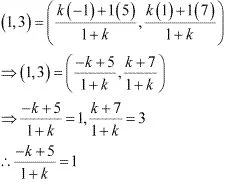⇒ -k + 5 = 1 + k
⇒ 2k = 4
⇒ k = 2
Thus, the line joining the points (-1, 1) and (5, 7) is divided by line  x + y = 4 in the ratio 1: 2.

15. Find the distance of the line 4x + 7y + 5 = 0 from the point (1, 2) along the line 2x – y = 0.

Solution

The given lines are
2x – y = 0 …(1)
4x + 7y + 5 = 0 …(2)
A (1, 2) is a point on line (1).
Let B be the point of intersection of lines (1) and (2).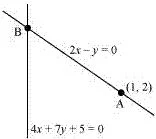On solving equations (1) and (2), we obtain x = 5/18 and y = -5/9 .
∴ Coordinates of point B are (-5/18 , -5/9).
By using distance formula, the distance between points A and B can be obtained as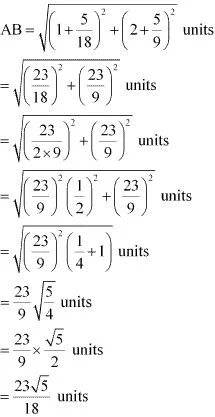Thus, the required distance is 23√5/18 units.

16. Find the direction in which a straight line must be drawn through the point (–1, 2) so that its point of intersection with the line x + y = 4 may be at a distance of 3 units from this point.

Solution

Let y = mx + c be the line through point (–1, 2).
Accordingly, 2 = m (–1) + c.
⇒ 2 = – m + c
⇒ c = m + 2
∴ y = mx + m + 2 …(1)
The given line is
x + y = 4 …(2)
On solving equations (1) and (2), we obtain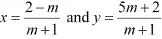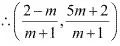is the point of intersection of lines (1) and (2).
Since this point is at a distance of 3 units from point (-1, 2), according to distance formula,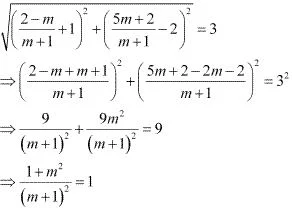⇒ 1 + m2 = m2 + 1 + 2m
⇒ 2m = 0
⇒ m = 0
Thus, the slope of the required line must be zero i.e., the line must be parallel to the x - axis.

17. The hypotenuse of a right angled triangle has its ends at the points (1, 3) and (−4, 1). Find the equation of the legs (perpendicular sides) of the triangle.

Solution

Let A(1,3) and B(−4,1) be the coordinates of the end points of the hypotenuse.
Now, plotting the line segment joining the points A(1,3) and B(−4,1) on the coordinate plane, we will get two right triangles with AB as the hypotenuse. Now from the diagram, it is clear that the point of intersection of the other two legs of the right triangle having AB as the hypotenuse can be either P or Q.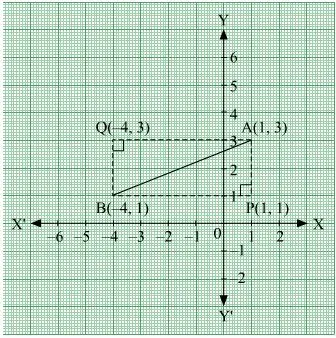Case 1: When ∆ APB is taken.
The perpendicular sides in ∆ APB are AP and PB.
Now, side PB is parallel to x-axis and at a distance of 1 units above x-axis.
So, equation of PB is, =1 or y−1= 0.
The side AP is parallel to y-axis and at a distance of 1 units on the right of y-axis.
So, equation of AP is =1 or x−1= 0.

Case 2: When ∆ AQB is taken.
The perpendicular sides in ∆ AQB are AQ and QB.
Now, side AQ is parallel to x-axis and at a distance of 3 units above x-axis.
So, equation of AQ is, = 3 or −3 = 0.
The side QB is parallel to y-axis and at a distance of 4 units on the left of y-axis.
So, equation of QB is = −4 or + 4 = 0.
Hence, the equation of the legs are :
=1, =1 or = −4, = 3

18. Find the image of the point (3, 8) with respect to the line x + 3y = 7 assuming the line to be a plane mirror.

Solution

The equation of the given line is
x + 3y = 7 …(1)
Let point B (ab) be the image of point A (3, 8).
Accordingly, line (1) is the perpendicular bisector of AB.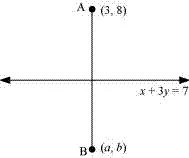Slope of AB = (b - 8)/(a - 3), while the slope of line (1) = -1/3
Since line (1) is perpendicular to AB,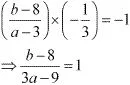⇒ b - 8 = 3a - 9
⇒ 3a - b = 1 ...(2)
Mid - point of AB =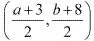The mid - point of line segment AB will also satisfy line (1).
Hence, from equation (1), we have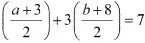⇒ a + 3 + 3b + 24 = 14
⇒ a+  3b = -13 ...(3)
On solving equations (2) and (3), we obtain a = -1 and b = -4.
Thus, the image of the given point with respect to the given line is (-1, -4).

19. If the lines y = 3x + 1 and 2y = x + 3 are equally inclined to the line y = mx + 4, find the value of m.

Solution

The equations of the given lines are
y = 3x + 1 …(1)
2y = x + 3 …(2)
y = mx + 4 …(3)
Slope of line (1), m1 = 3
Slope of line (2),
Slope of line (3), m= m
It is given that lines (1) and (2) are equally inclined to line (3). This means that
the angle between lines (1) and (3) equals the angle between lines (2) and (3).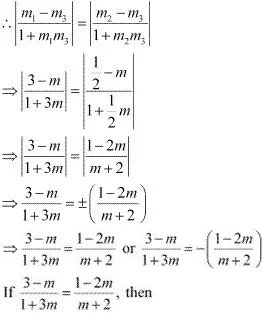(3 - m)(m +2) = (1 - 2m)(1 + 3m)
⇒ -m2 + m + 6 = 1 + m -6m2
⇒ 5m2 + 5 = 0
⇒ (m2 + 1) = 0
⇒ m = √-1 , which is not real
Hence, this case is not posible.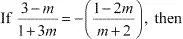⇒ (3 - m)(m + 2) = -(1 - 2m)(1 + 3m)
⇒ -m2 + m + 6 = -(1 + m - 6m2
⇒ 7m2 - 2m - 7 = 0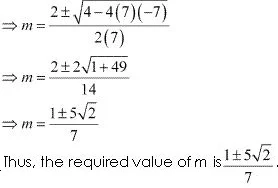20. If sum of the perpendicular distances of a variable point P (x, y) from the lines x + y – 5 = 0 and 3x – 2y+ 7 = 0 is always 10. Show that P must move on a line.

Solution

The equations of the given lines are
x + y – 5 = 0 …(1)
3x – 2y + 7 = 0 …(2)
The perpendicular distances of P (x, y) from lines (1) and (2) are respectively given by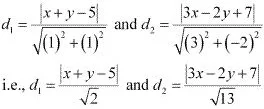It is given that d1 + d2 = 10.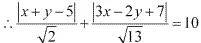⇒ √13|x + y - 5| + √2|3x - 2y + 7| - 10√26 = 0
⇒ √13(x + y - 5) + √2(3x - 2y + 7 )-10√26 = 0
[Assuming (x + y - 5) and (3x - 2y + 7) are positive]
⇒ √13x + √13y - 5√13 + 3√2x - 2√2y + 7√2 - 10√26 = 0
⇒ x(√13 + 3√2) + y(√13 - 2√2) + 7(√2 - 5√13 - 10√26) = 0 , which is the equation of a line.
Similarly, we can obtain the equation of line for any signs of (x + y - 5) and (3x - 2y + 7).
Thus, point P must move on a line.

21. Find equation of the line which is equidistant from parallel lines 9x + 6y – 7 = 0 and 3x + 2y + 6 = 0.

Solution

The equations of the given lines are
9x + 6y – 7 = 0 …(1)
3x + 2y + 6 = 0 …(2)
Let P (h, k) be the arbitrary point that is equidistant from lines (1) and (2). The perpendicular distance of P (h, k) from line (1) is given by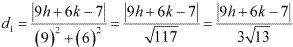The perpendicular distance of P (h, k) from line (2) is given by: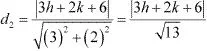Since P(h, k) is equidistant from lines (1) and (2), d1 = d2.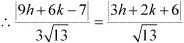⇒ |9h + 6k -7| = 3|3h + 2k + 6|
⇒ |9h + 6k -7| = ±3|3h + 2k + 6|
⇒ 9h + 6k - 7 = 3(3h + 2k + 6) or 9h + 6k - 7 = -3(3h + 2k + 6)
The case 9h + 6k -7 = 3(3h + 2k + 6) is not possible as
9h + 6k - 7 = 3(3h + 2k + 6)
⇒ -7 = 18 (which is absurd)
∴ 9h + 6k - 7 = -3(3h + 2k + 6)
9h + 6k - 7 = -9h - 6k - 18
⇒ 18h + 12k + 11 = 0
Thu, the required equation of the line is 18x + 12y + 11 = 0.

22. A ray of light passing through the point (1, 2) reflects on the x-axis at point A and the reflected ray passes through the point (5, 3). Find the coordinates of A.

Solution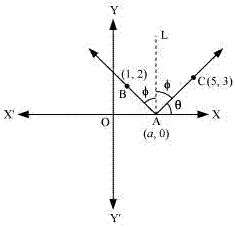Let the coordinates of point A be (a, 0) .
Draw a line (AL) perpendicular to the x - axis.
We know that angle of incidence is equal to angle of reflection. Hence, let
∠BAL = ∠CAL = Φ
Let ∠CAX = θ
∴∠OAB = 180° – (θ + 2Φ) = 180° – [θ + 2(90° – θ)]
= 180° – θ – 180° + 2θ
= θ
∴∠BAX = 180° – θ
Now, slope of line AC = (3 - 0)(5-a)
⇒ tan θ = 3/(5 - a) ...(1)
Slope of line AB = (2-0)/(1 - a)
⇒ tan (180° - θ) = 2/(1 - a)
⇒ - tan θ = 2/(1 -a)
⇒ tan θ = 2/(a - 1)  ...(2)
From equations (1) and (2), we obtain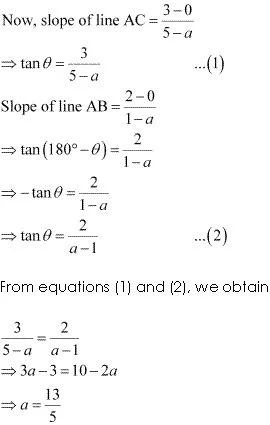Thus, the coordinates of point A are (13/5, 0).

23. Prove that the product of the lengths of the perpendiculars drawn from the points
[√(a2 - b2), 0] and [(-√a2  - b2), 0] to the line (x/a) cosθ + (y/b)sinθ = 1 is b2 .
Solution
The equation of the given line is
(x/a) cosθ + (y/b)sinθ = 1
Or, bx cosθ + ay sinθ - ab = 0 ...(1)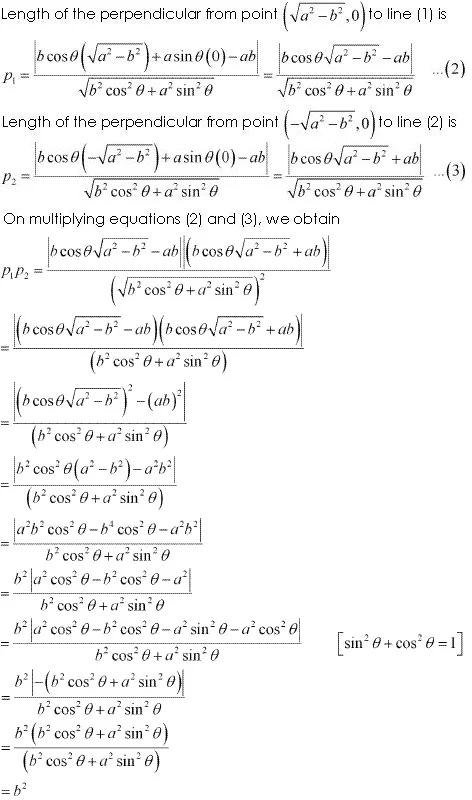Hence, proved.

24. A person standing at the junction (crossing) of two straight paths represented by the equations 2x – 3y+ 4 = 0 and 3x + 4y – 5 = 0 wants to reach the path whose equation is 6x – 7y + 8 = 0 in the least time. Find equation of the path that he should follow.
Solution

The equations of the given lines are
2x – 3y + 4 = 0 …(1)
3x + 4y – 5 = 0 …(2)
6x – 7y + 8 = 0 …(3)
The person is standing at the junction of the paths represented by lines (1) and (2).
On solving equations (1) and (2), we obtain x = -1/17 and y = 22/17.
Thus, the person is standing at point (-1/17, 22/17).
The person can reach path (3) in the least time if he walks along the perpendicular line to (3) from point (-1/17, 22/17).
Slope of the line perpendicular to line (3)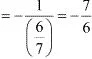The equation of the line passing through and having a slope of -7/6 is given by
(y - 22/17) = -7/6 (x + 1/17)
⇒ 6(17y - 22) = -7(17x + 1)
⇒ 102y - 132 = -119x - 7
⇒  119x + 102y = 125
Hence, the path that the person should follow is 119x + 102y = 125.